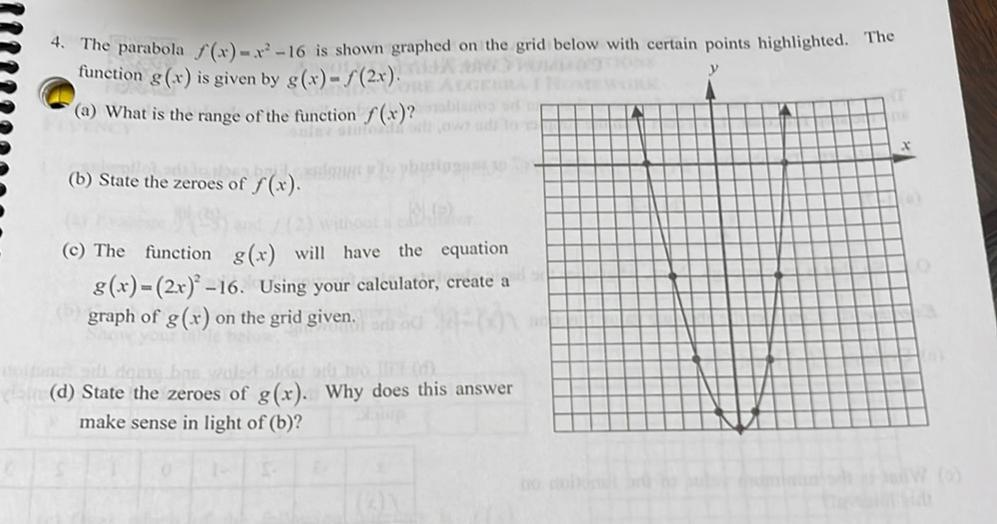Question:

# y 4. The parabola f (x) ----16 is shown graphed on the grid below with certain points highlighted. The function g(x) is given byy 4. The parabola f (x) ----16 is shown graphed on the grid below with certain points highlighted. The function g(x) is given by s(r) - | (2x) (a) What is the range of the function f(x)? IN x (b) State the zeroes of f(x). eroes of () (©) The function g(x) will have the equation 8(x) = (2x) –16. Using your calculator, create a graph of g(x) on the grid given. (d) State the zeroes of g(x). Why does this answer make sense in light of (b)?